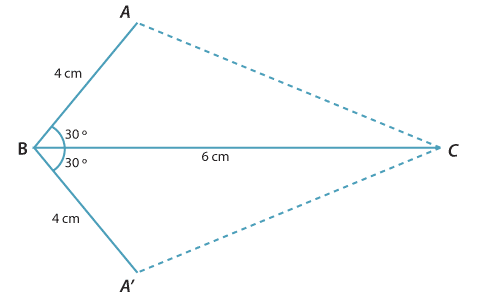#### The SAS congruence test

The three triangles on page 4 showed that when only two sides of a triangle are specified, there is nothing to stop those two sides flapping about. The other way to stop the two sides flapping is to specify the angle between them. This angle between the specified sides is called the included angle. The included angle can be any angle between $$0\,^{\circ}$$ and $$180\,^{\circ}$$.

#### Constructing a triangle given two sides and the included angle

To illustrate this, let us construct a triangle $$ABC$$ in which

$$AB = 4$$ cm$$BC = 6$$ cm$$B = 30\,^{\circ}$$ (This is the included angle.)

$$\triangle A'BC$$ is the reflection of $$\triangle ABC$$ in $$BC$$.Any triangle with side lengths 4 cm and 6 cm and included angle $$30\,^{\circ}$$ is congruent to triangle $$ABC$$.